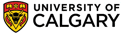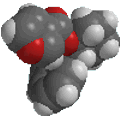Chapter 13: Spectroscopy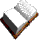Different types of Hydrogen

This may seem a trivial concept, but the idea of establishing the number of kinds of hydrogens (or other atoms such as C) is a very important useful concept, especially in NMR spectroscopy where for example, different types of H (or other atoms) typically each give rise to a different signal. The idea is developed below for H atoms, but can be extended to other types of atom.

What do we mean?
A hydrogen atom is "different" to another hydrogen atom if it is not in an identical environment (location) to the other hydrogen. This could mean it is attached to a different type of atom (e.g. compare CH vs OH, or sp3 CH vs sp2 CH), or due to the number of adjacent H (e.g. CH3- vs -CH2- ) or just at a different point in a chain (e.g. compare the H in the methylene (CH2) groups in CH3CH2CH2OH which has 4 types of H.

Counting different types of Hydrogen
There are three methods that can be used to count the number of kinds of H (each achieve the same result).  You will probably find it best to master the first of these methods and over time you will find yourself moving towards the 3rd method)

1. Substitution method (simplest but slowest)
The idea is that you replace each H in the molecule in turn with a "dummy" atom (for example a -Cl) to see if you get a different product (i.e. one that will require a different name). Each new product, indicates a different type of  H. This idea is related to the radical chlorination of alkanes where some of each possible product is usually obtained.

For example:

Qu : If you remove one H atom from chloroethane and substitute it for another Cl atom, how many different molecules can you make ?
Ans : Two products, 1,1-dichloroethane and 1,2-dichloroethane. Hence there are two types of H in chloroethane

2. Verbal description
The verbal method requires that you describe the position of each H within the molecule.  If you need to use different words to describe two H atoms, then they represent different types of H.

For example:

• an -OH is different to a -CH (based on what they are attached to)
• a -CH3 is different to a -CH2- (because the number of H at each C are different)
• an sp3 C-H is different to an sp C-H.
• Other differences could be position on a chain, across a ring or double bond etc.

3.
Symmetry (most difficult but fastest)
The symmetry method is the most sophisticated but the quickest method and requires that you look for mirror planes, rotation axes or inversion centers that interchange H atoms.  H atoms that can be interchanged by symmetry are equivalent to each other.

 CAUTION Remember that rotation about σ-bonds produces different conformations only and not different molecules. Remember to consider the three dimensional nature of the molecule

QUESTIONS

 How many different types of H are there in each of the following hydrocarbons: propane, 2-methylbutane and propene ? ANSWER ANSWER ANSWER
 How many types of H are there in bromomethane ?ANSWER

Terminology
 Homotopic Replacement of the groups gives the same product For example, it doesn't matter which of the H atoms in bromomethane is replaced with chlorine, we always get bromochloromethane. Hence these three H are said to be homotopic. Homotopic H always give the same NMR signal. Enantiotopic Replacement of the groups gives enantiomers Consider the H atoms in the methylene group in bromoethane. If we replace one of those H with a Cl, we create a chirality center. Therefore depending on which of the two H is replaced, we get one enantiomer or the other. Hence these two H are said to be enantiotopic.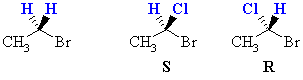Enantiotopic H give the same NMR signal in an achiral environment (normal NMR conditions) Diastereotopic Replacement of the groups gives diastereomers. Consider the H atoms in the methylene group in 1-bromo-1-chloropropane. There is already a chirality center at C1.  If we replace one of those H with a Cl, we create a new chirality center.  Therefore depending on which of the two H is replaced, we get one diastereomer or the other. Hence these two H are said to be diastereotopic.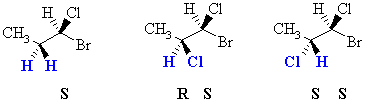Diastereotopic H : are different and in principle they give rise to different signals and hence they can couple to each other (see later).

QUESTIONS

• How are the H in bromoiodomethane related ? ANSWER
• How are the H in the methyl group in bromoethane related ? ANSWER© Dr. Ian Hunt, Department of Chemistry